Interesting script?
So post a link to it -
let others appraise it
You liked the script? Try it in the MetaTrader 5 terminal# All In One Divergence - indicator for MetaTrader 4

Petr
Views:
100135
Rating:
Published:
2017.09.25 08:01
Updated:
2021.06.28 07:32

This indicator is based on code from the original author Petr, published by Aleh Sasonka.

• Inputs to enable/disable each alert type separately.
• Dropdown list of 30 indicators with real names instead of numbers.
• Signal buffer (Indicator output value).
• Indicator is compatible with Universalis MT4, you can import it, create your own automated strategy and backtest with high precision (step-by-step guide).

• Osc=29 - the number of the oscillator.
• TH=true - bearish divergence.
• TL=true - bullish divergence.
• trend=true - support/resistance lines.
• convergen = true - hidden divergence.
• Complect = 1 - the distinguishing index of the indicator on the chart.
• BackSteph = 0 - number of steps back h.
• BackStepl = 0 - number of steps back l.
• BackStep = 0 - number of steps back.
• qSteps = 1 - number of displayed steps, no more than three.
• LevDPl = 5 - DeMark level points (2 = the central bar will be above (below) the two bars on the left).
• LevDPr = 1 - DeMark level points (2 = the central bar will be above (below) the two bars on the right).
• period = 8 - period for iADX, iATR, iBearsPower, iBullsPower, iCCI, iDeMarker, iForce, iMomentum, iMFI, iRVI, iStdDev, iRSI, iRSI, iWPR.
• ma_method = 0 - averaging method for iStochastic, iStdDev, iForce.
• ma_shift = 0 - MA shift for iStdDev.
• applied_price = 4 - applied price for iRSI, iRSI, iStdDev, iOBV, iOsMA, iMACD, iMomentum, iForce, iCCIiBullsPower, iBearsPower, iADX.
• mode=0 - line index for iStochastic, iRVI, iMACD, iADX.
• fast_ema_period = 12 - fast MA period for iOsMA, iMACD.
• slow_ema_period = 26 - slow MA period for iOsMA, iMACD.
• signal_period = 9 - signal line period for iOsMA, iMACD.
• Kperiod = 13 - K line period for iStochastic.
• Dperiod = 5 - D line period for iStochastic.
• slowing = 3 - slowing for iStochastic.
• price_field = 0 - applied price for iStochastic.
• showBars = 1000 - if = 0, the indicator is displayed for the entire chart.
• Trend_Down = true - resistance lines.
• Trend_Up = true - support lines.
• TrendLine = true - support/resistance lines.
• HandyColour = true.
• Highline = Red - HL channel color.
• Lowline = DeepSkyBlue - LL channel color.
• ChannelLine = false - channels parallel to trend lines.
• Trend = 0 - 1 = only for UpTrendLines, -1 = only for DownTrendLines, 0 = for all TrendLines.
• Channel = false - channels.
• Regression = true - Linear Regression Channel, false - Standard Deviation Channel.
• RayH = true - ray for the High channel.
• RayL = true - ray for the Low channel.
• ChannelH = Red - color of the High channel.
• ChannelL = DeepSkyBlue - color of the Low channel.
• STD_widthH = 1.0 - deviation size for the CHANNEL High object.
• STD_widthL = 1.0 - deviation size for the CHANNEL Low object.
• comment = false - display comments.
• code = 159 - character code of the Wingdings font to display the extremums.
• SellLimit = false - SellLimit levels.
• SellStop = false - SellStop levels.

### Examples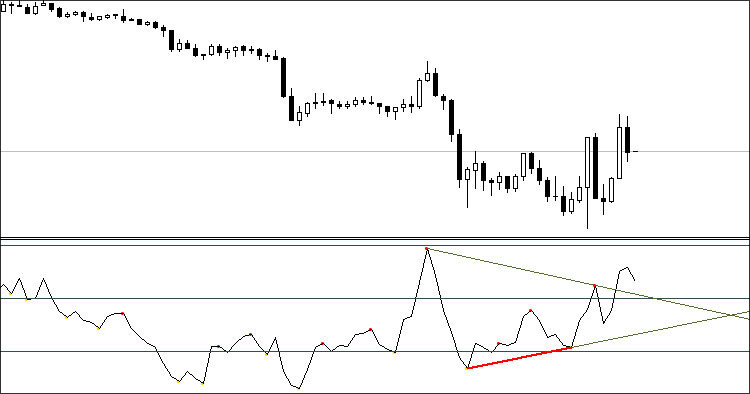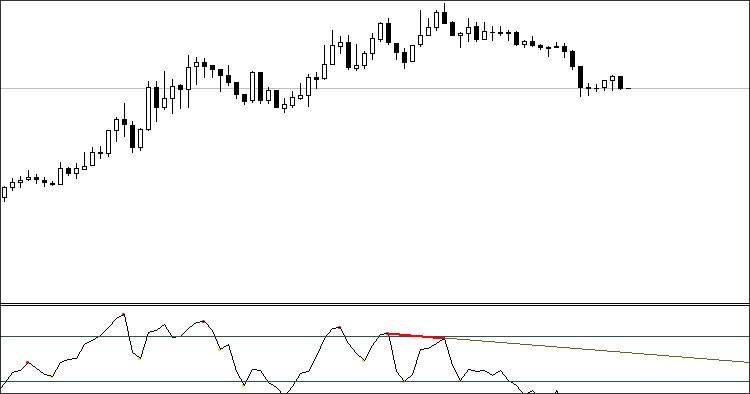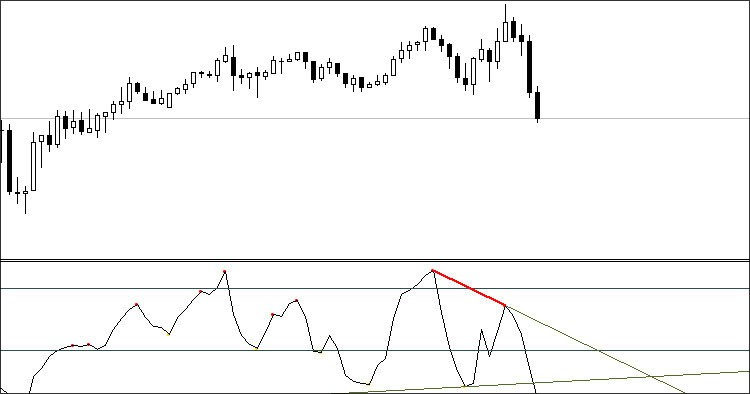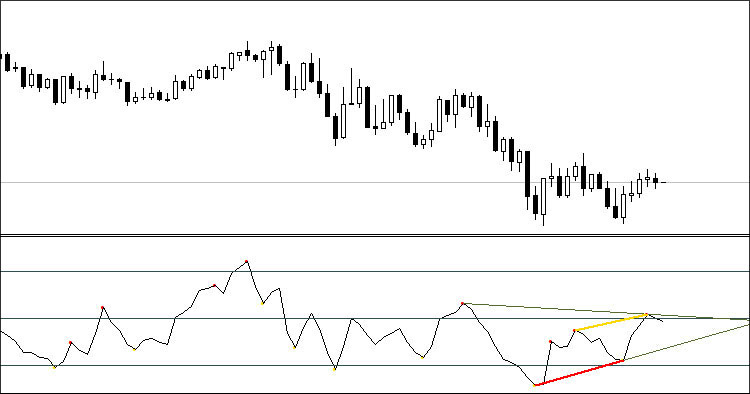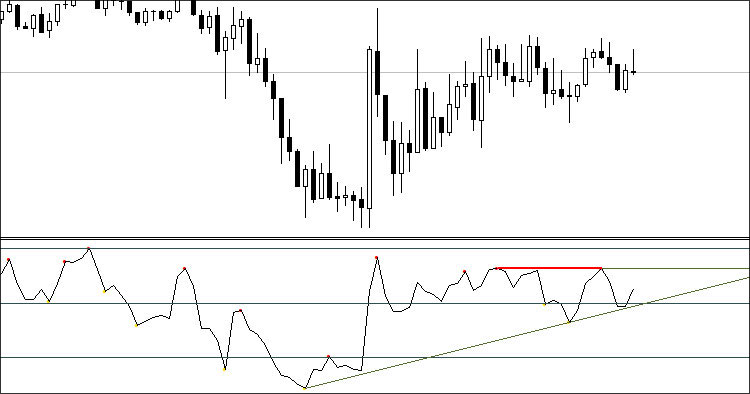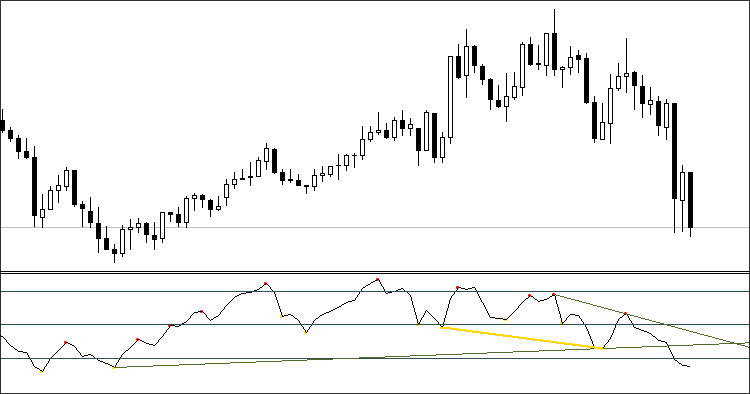### Recommendations

• Change the Complect parameter when attaching more than one indicator to the chart.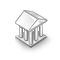Hidden StopLoss and TakeProfit System

A class system to represent a hidden stop loss and take profit system, combined with 4 example files showing the use of the classes.S4T_DaysOfTheWeek

The indicator marks the days of the week with a colored histogram in the chart sub-window.Simpler Trend

Get a grasp of the current trend by candle colors.Consecutive Candles with Stochastic Filter Indicator

Shows when two or three consecutive bull or bear candles of a certain size print after qualifying the stochastic level of the initial candle in the series. New in version 3 /* Changelog 2-4-19 Added 50% line to indicator box - Ignored changing the indicator lines individually since mql does not have a built-in language convention to create this change would require too much additional research and effort. Fixed error with the DodgerBlue lines not showing, the problem was due to using DodgerBlue instead of clrDodgerBlue in the code to indicate color, should be fixed now. */